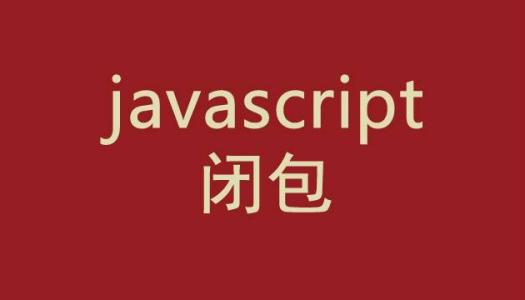﻿ JavaScript闭包实例讲解 – 码云笔记

# JavaScript闭包实例讲解

113℃

[隐藏]## 一、函数内部直接读取全局变量，代码如下：

```var n = 999;

function f1() {

}

fi()   //999```

## 二、函数的外部是无法读取函数内部的局部变量

```function f1(){
var n=999;
}

```function f1(){
n=999;
}

f1();

## 三、如何从外部读取局部变量？

```function f1(){

var n=999;

function f2(){
}

}```

```function f1(){

var n=999;

function f2(){
}

return f2;

}

var result=f1();

result(); // 999```

## 四、那么闭包的概念是什么呢？

```function f1(){

var n=999;

function f2(){
}

return f2;

}

var result=f1();

result(); // 999

result(); // 1000```

HTML代码：

```<ul id="list">

<li>第1行</li>

<li>第2行</li>

<li>第3行</li>

<li>第4行</li>

<li>第5行</li>

<li>第6行</li>

</ul>```

JS代码：

```var \$li = document.getElementById("list").getElementsByTagName("li");

var i=0;

for(;i < \$li.length;i++){

\$li[i].onclick = function(){

console.log(i);

}

}```

```var \$li = document.getElementById("list").getElementsByTagName("li");

function A(){

var i=0;

for(;i < \$li.length;i++){

B(i);

}

}

function B(index){

\$li[index].onclick = function(){

console.log(index);

}

}

A();```

```var i=0;

for(;i < \$li.length;i++){

function test(){

console.log(i);

}

}

test();//输出的是6```

```function A(){

for(var i=0;i < \$li.length;i++){

B(i);

}

}

function B(index){

console.log(index);

}

A();//输出的是0，1,2,3,4,5```

## 五、使用闭包的注意点

1）由于闭包会使得函数中的变量都被保存在内存中，内存消耗很大，所以不能滥用闭包，否则会造成网页的性能问题，在IE中可能导致内存泄露。解决方法是，在退出函数之前，将不使用的局部变量全部删除。

2）闭包会在父函数外部，改变父函数内部变量的值。所以，如果你把父函数当作对象（object）使用，把闭包当作它的公用方法（Public Method），把内部变量当作它的私有属性（private value），这时一定要小心，不要随便改变父函数内部变量的值。

```function A(){

for(var i=0;i < \$li.length;i++){

B(i);

}

}

function B(index){

console.log(index);

}

A();//输出的是0，1,2,3,4,5

B = null;```